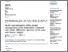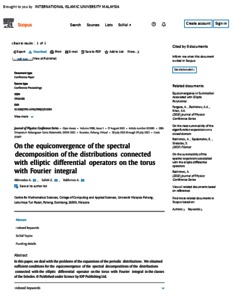# On the equiconvergence of the spectral decomposition of the distributions connected with elliptic differential operators on the torus with fourier integral

Akhmedov, Abdulkasim and Salleh, Zuki and Rakhimov, Abdumalik (2021) On the equiconvergence of the spectral decomposition of the distributions connected with elliptic differential operators on the torus with fourier integral. In: Simposium Kebangsaan Sains Matematik ke-28 (SKSM28), 28-29 July 2021, Centre for Mathematical Sciences Universiti Malaysia Pahang..PDF (IOP conference series) - Published Version
Restricted to Repository staff onlyPDF (slide presentation) - Presentation
Restricted to Repository staff onlyPreview
PDF (scopus) - Supplemental Material

## Abstract

The problems of engineering sciences can be modelled using equations of mathematical physics. Mathematical models for many systems that are encountered in engineering, physics, and other applied sciences are often developed by applying various laws that describe the conservation of mass, momentum, and energy. These models are usually given as a single or set of ordinary or partial differential equations along with appropriate initial and boundary conditions which apply over the rectangular region. Solution of these equations using appropriate analytical methods provides local numerical values for the dependent variables of interest, such as fluid velocity, pressure, species concentration, temperature, force and electric potential.

Item Type: Conference or Workshop Item (Invited Papers) 6741/91448 equconvergence, fourier intergal, multiple series, summation methods Q Science > QA Mathematics > QA300 Analysis Kulliyyah of Engineering > Department of Science Professor Abdumalik Rakhimov 07 Oct 2021 10:21 07 Oct 2021 10:24 http://irep.iium.edu.my/id/eprint/91448View Item